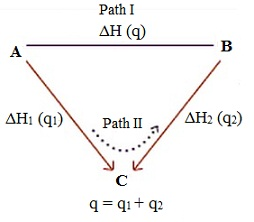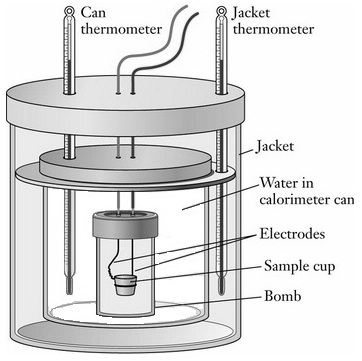#### Enthalpy changes in different processes and reactions, Chemistry tutorial

Introduction:

Thermochemistry examines the relationship between the chemical reactions and energy changes comprising heat. It was born out of the practical problem of cannon making and nowadays continues to play a significant role in nearly each and every facet of the chemistry. The practical applications of Thermochemistry comprise the growth of alternative fuel sources, like fuel cells, hybrid gas-electric cars and gasoline supplemented with ethanol. On a primary level, Thermochemistry is as well significant as the forces holding molecules or ionic compounds altogether are associated to the heat evolved or absorbed in the chemical reaction. Thus, chemists are interested in the Thermochemistry of each and every chemical reaction, whether it is the solubility of lead salts in drinking water or metabolism of the glucose.

Chemical reactions generally accompany the formation and absorption of energy in the form of heat. The stream of chemical science dealing with the study of heat and energy changes is termed as thermodynamics. The heat changes in a specific system can be studied by employing the device termed as calorimeter.

Calorimetry is basically derived from the Latin word 'calor' that signifies heat, and the Greek word 'metry' signifies to measure, the heat in the system. The latest advances in the Calorimetry and electronics help the user to maintain the samples beneath proper conditions that can be simply analyzed.

The Scottish physician Joseph Black, was the former to recognize the difference between heat and temperature, and is stated to be the 'Founder of Calorimetry'. It is noted that the credits for modern methods of Calorimetry is given to French chemist Pierre Eugene Berthelot (1827-1907). He studied the problems related with the heat measurement and constructed the first modern calorimeter. The words exothermic and endothermic reactions were introduced to explain heat reactions and are as well his contributions to the chemistry.

Usually the reactions occurring in the chemical sciences are breaking and making of chemical bonds. This is accompanied through some heat effects. The formation of chemical bonds discharges energy in the form of heat and therefore termed as the exothermic reaction. The reaction that is accompanied absorption of heat is termed as endothermic reaction. Calorimetry is scientific term dealing by the changes in energy of the system by measuring the heat exchanged by the surroundings. In a wider sense it is defined to find out the heat released or absorbed in the chemical reaction. The calorimeter is a device designed to measure heat of reaction or physical changes and heat capacity. The device can be sophisticated and expensive or simple and cheap.

A calorimeter comprises of two vessels, outer vessel and an inner vessel. The space between these vessels acts as the heat insulator and thus there is very little heat exchange in between the inner and outer vessels. Thermometer evaluates the temperature of the liquid in the inner vessel. The stirrer functions in such a manner to stir the liquid to distribute the heat in the whole vessel. The fibre rings in the calorimeter assists to hold the inner vessel hanging in the center of the outer vessel. It as well consists of an insulating cover or lid with holes for attaching the stirring rod and thermometer.

Hess's law of constant heat summation:

G.H. Hess introduced a law concerning the heats or enthalpies of reaction in the year 1840 termed as the Hess's law. This law defines that the heat change in a specific reaction is similar whether it occurs in one step or several steps.

For illustration: a reactant 'A' changes to a product 'B' in one step and the heat change throughout this method is dH. If the reaction is taken out in two steps where 'A' first changes to 'C' an intermediate phase and then 'C' changes to 'B' in the given step then let the heat change throughout the formation of 'A' to 'C' be dH1 and that from 'C' to 'B' be dH2. From Hess's law the heat change for the reaction is represented as:

dH = dH1 + dH2Fig: Hess's law of constant heat summation

This signifies that the amount of heat absorbed or evolved in a chemical reaction depends only on the energy of initial reactants and the final products. The heat change is independent of the path or the way in which the change has occurred.

The formation of carbon-dioxide from carbon and oxygen can be described as follows. Carbon can be transformed into carbon-dioxide in two ways. At first, solid carbon joins with adequate amount of oxygen to form CO2. The similar reaction whenever carried in presence of lesser amount of oxygen provides carbon monoxide which then gets transformed to CO2 in step two in the presence of oxygen.

Path I: C(s) + O2 (g) → CO2 (g) ΔH = - 393.5 KJ

Path II: C(s) + 1/2 O2 (g) → CO (g) ΔH1 = - 110.5 KJ

CO (g) + 1/2 O2 (g) → CO2 (g) ΔH2 = - 283.0 KJ

--------------------------------------------------------

C(s) + O2 (g) → CO2 (g) ΔH = - 393.5 KJ

dH = dH1 + dH2

Therefore, one can conclude that Thermochemical equations can be added, subtracted or multiplied similar to algebraic equations to get the desired equation.

Enthalpy changes in different processes and reactions:

Enthalpy of Combustion:

The enthalpy of combustion of a compound is the enthalpy change at normal pressure and at constant temperature accompanying the complete combustion of one mole of the compound. It is represented by dHc. Combustion here signifies the burning of the given compound to the highest oxides of the constituent elements in the presence of surplus of oxygen.

For illustration: the enthalpy of combustion of benzene at 298 K is the enthalpy change of the reaction.

C6H6 (l) + 15/2 O2 (g) → 6CO2 (g) + 3H2O (l) ΔH = - 3268KJ

Therefore, dHcomb C6H6 (l) = - 3268 kJmol-1.

The enthalpy of combustion is usually obtained experimentally. For conditions, where it is not possible to measure it experimentally, it is evaluated from the enthalpies of formation of the different compounds comprised in the process.

Enthalpy of Neutralization:

The reaction in which the acid and base react to give a salt and water is termed as neutralization reaction. Neutralization reactions are exothermic in the nature. The heat change whenever one gram equivalent of an acid is fully neutralized via a base or vice-versa in dilute solution is termed as heat of neutralization.

Examples of heat of neutralization are:

=> Neutralization of HCl with NaOH:

HCl (aq) + NaOH (aq) → NaCl (aq) + H2O (l) ΔH = - 57.1 KJ

=> Neutralization of CH3COOH with NaOH:

CH3COOH (aq) + NaOH (aq) → CH3COONa + H2O (l)

dH = - 55.9 kJ

Enthalpy of solution (dHsol):

Whenever a solute is dissolved in a solvent a solution is made. Throughout the dissolution of a solute in any solvent, some amount of heat is either evolved or absorbed. Such heat changes beneath constant pressure conditions are termed as the enthalpy of solution. The change in enthalpy whenever one mole of a solute is dissolved in the specified quantity of a solvent at a given temperature is termed as enthalpy of solution.

To avoid the quantity of solvent, heat of solution is generally stated for an infinite dilute solution. Therefore, heat of solution at infinite dilution is the heat change whenever one mole of a substance is dissolved in such a big quantity of solvent in such a manner that further dilution doesn't provide any further heat change.

For illustration, the dissolution of sodium chloride:

NaCl (s) + aq → NaCl (aq) ΔH = ΔHsol = + 5.0 KJ

Here 'aq' symbolizes aqueous meaning a large surplus of water.

For substances that dissolve with the absorption of heat (that is, endothermic), the enthalpy of solution is positive whereas for the substances which dissolve by discharging heat (that is, exothermic), the enthalpy of solution is negative.

For illustration, if KCl is dissolved in water, heat is absorbed. Therefore, the enthalpy of solution of KCl is positive. For a 200 times dilution

(Water: KCl = 200: 1), the enthalpy change throughout the process,

KCl (s) + 200 H2O → KCl (aq) ΔH = + 18.6KJ

Therefore, the enthalpy of solution of KCl at a dilution of 200 is 18.6 kJ mol-1. The dissolution of CaCl2(s) in water is the exothermic process. Therefore, the enthalpy of solution of calcium chloride (CaCl2) is negative. At a dilution of 400, the enthalpy change for the reaction,

CaCl2 (s) + 400 H2O → CaCl2 (aq) ΔH = - 75.3 KJ

Therefore, the enthalpy of solution of CaCl2(s) at a dilution of 400 is - 75.3 KJ

Experimental determination of enthalpy of combustion:

Calorimeters are employed for finding out the enthalpy changes in different processes. As a matter of fact, Calorimetry is the most significant experimental method in thermodynamics and a lot has been done to make it very sensitive. At present, there are calorimeters which can measure heat and temperature changes precisely.

The enthalpy of combustion is found out employing the Bomb calorimeter (figure shown below):Fig: Bomb calorimeter

The method comprises the combustion of a definite amount of substance in an inner vessel termed as the Bomb. The bomb is positioned within a definite quantity of water taken in an outer vessel that is completely insulated. The temperature readings of water in it are before and after the combustion, by employing a sensitive thermometer. From the heat capacity of the calorimeter, the amount of substance taken and the temperature readings, the enthalpy of combustion can be computed in general; by using adiabatic calorimeters the enthalpies of reactions can be determined.

Tutorsglobe: A way to secure high grade in your curriculum (Online Tutoring)